## Introduction

Artificial effective media are attractive because of the fantastic applications such as super lens and electromagnetic invisibility1,2. However, the inevitable loss due to their strongly dispersive nature is one of the fundamental challenges preventing such applications from becoming a reality. Recently, the discussions of incorporating active elements into artificial media to compensate loss and potential bandwidth have attracted more and more interests3,4,5,6,7,8,9,10,11,12,13. However, intense controversies on the causality issue of artificial active media still exist. Up to now, on the assertion whether there is a theoretical stable state for the simultaneous existence of negative refractive index (NRI) and net gain, physicists still have opposite opinions14,15,16,17,18,19. In optics, a diverse family of ‘gain media’, primarily based on doped crystalline, semiconductors, dyes and gases, have been widely used in laser technologies20,21,22,23,24. Simulations have shown that by placing optical gain media (that is, optically pumped laser dyes) in fishnet metamaterial cells, the resulting optical ‘gain metamaterial’ is able to exhibit an NRI in a narrow bandwidth without violating causality4,7. The significance of this conception is that although this artificial gain medium still functions as a laser amplifier, thus compensating or even overcompensating the intrinsic loss of traditional metamateirals, it inherits the theoretical superiorities of metamaterials, being able to provide arbitrarily controlled constitutive parameters not found in nature5. Therefore, ‘gain metamaterials’ have the realistic potential in implementing metamaterial applications such as perfect lenses and invisibility cloaks, where intrinsic losses fundamentally plague their realizations. However, to our knowledge, although significant loss compensation for a fishnet metamaterial at optical frequency has been reported8, metamaterial with NRI and net gain has not been reported so far.

In this study, we experimentally demonstrate active metamaterial with simultaneous gain and negative index of refraction in microwave regime. We discuss the theory and implementation of an artificial microwave gain medium based on negative-resistance devices (that is, external energy pumping from direct current (DC) power supply). We derive the condition for achieving such a medium from the electromagnetic energy conservation in causal media. Equivalent circuit analysis, simulations and experimental measurements show that a fabricated sample consisting of traditional metamaterial building blocks with embedded microwave tunnel diodes (TDs) indeed exhibits a band-limited Lorentzian dispersion with overcompensation of loss and NRI. Our work provides experimental evidence to the discussion of the causality of negative-index gain medium, proposing a potential solution for the critical challenge current metamateiral technology faces in practical applications.

## Results

### Homogenization of effective gain medium

In Maxwell electromagnetic (EM) theory, the energy conservation of an EM wave propagating in a causal, naturally existing medium is described by Poynting’s theorem25: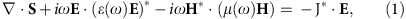where ε(ω) and μ(ω) represent the causally dispersive permittivity and permeability, respectively, ·S is the divergence of complex Poynting vector, whose real part represents the total power flowing out an infinitesimally small closed volume, which is always negative for lossy passive media. The term −JE in equation (1) may consist of a conductive current density Jc(ω)=σc(ω)E(ω), where σc(ω) is the causally dispersive conductivity, and a free current density Js. It should be noted that Js=0 in any passive medium. However, if Js can be artificially provided homogeneously in the medium, and it can be controlled by the incident field, that is, Js(ω)=σs(ω)E(ω), where σs(ω) is an effectively ‘negative’ conductance that is independent of E(ω), equation (1) can be rewritten as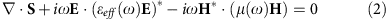where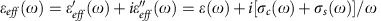can be regarded as the effective permittivity of a new artificial medium. For a plane wave propagating in such an effective medium, assume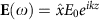and H(ω)=ŷE0eikz/η, where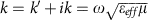and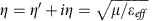are the complex wave number and wave impedance, respectively. Substitute E(ω) and H(ω) into equation (2), we obtain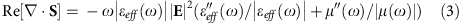For a sufficiently negative σs(ω),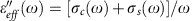can be negative, resulting in a positive Re[·S]. In this case, the effective medium described by εeff(ω) can be macroscopically considered as a gain medium, providing a spatially uniform amplification to incident waves. It is worth noting that the dispersion of εeff(ω) is a linear superposition of the conventional complex permittivity ε(ω)+c(ω)/ω and s(ω)/ω, meaning that the causality of εeff(ω) fully depends on the dispersion of σs(ω). That said, from energy conservation (Poynting’s theorem) point of view, if σs(ω) can be physically obtained, εeff(ω) will consequently satisfy causality.

It is seen that the key to implement an artificial gain medium is to obtain a uniform spatial distribution of a negative conductance σs(ω) (or resistance). In microwave regime, this can be achieved by embedding negative-resistance devices, such as TDs, into conventional sub-wavelength metamaterial unit cells, as shown in Fig. 1. TDs are quantum tunnelling semiconductor devices capable of offering a negative differential resistance (NDR) at microwave frequencies6,26. This NDR can be equivalently considered as a negative resistance −Rd, acting as a current source linearly controlled by an applied voltage. Similar NDR device was used to improve the quality factor of a split ring resonator (SRR) by partially compensating its loss11. In Fig. 1a, we show examples of embedded TD in sub-wavelength wire and SRR cells, which are the basic building blocks for constructing metamaterials.

For the wire array under a z-polarized plane wave incidence, the voltage across the sub-wavelength wires can be expressed as U=Ezd=RI+(−iωL)I+I/(−iωC)+Ud, where I is the induced current, R and L are the total parasitic resistance and inductance, respectively, (including mutual inductance between adjacent wires) of the wire27,28, C is the total series capacitance caused by the open ends of the wire and the gap used to mount the TD and Ud=−IRd is the voltage across the TD. Then, the volume current density Jv in each cell can be homogenized as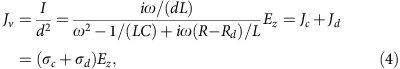where d is the periodicity of the cell. According to equation (2), εeff can be described by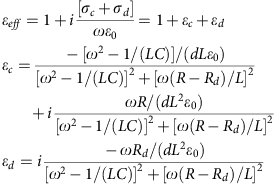Note that the signs of the imaginary parts of εc and εd depend on the positive parasitic resistance R and the negative resistance −Rd of the TD, respectively. In Fig. 1b, we plot the εeff derived above. Relevant parameters are d=0.02 m, R=30 Ω, L=5 nH and C=1.25 pF, where L and C are determined based on refs 27, 28. It is seen that when −Rd is −10 Ω, although the positive imaginary part of εc is compensated by εd, the overall imaginary part of εeff remains positive so that the overall effective medium has a loss. However, when −Rd is −50 Ω, overcompensation occurs and εeff effectively has a negative imaginary part so that the overall effective medium has a gain. According to ref. 3, the real and imaginary parts of εeff satisfy the Krammers–Kronig relations defined for active media, implying that this εeff obeys causality.

In a similar way, we can also derive the effective permeability with a potentially negative imaginary part for the SRR array embedded with TDs as shown in Fig. 1a. Details about this derivation and the corresponding equation of μeff can be found in Methods section.

For a metamaterial comprising such wire and/or SRR cells, when −Rd is chosen appropriately so that the loss of the metamaterial can be overcompensated, an effective microwave gain medium with a unique frequency dispersion determined by the ‘active’ resonance of these sub-wavelength resonators, including a NRI, can be realized.

### Full-wave simulation

For demonstration, we design a new single-layer cell that combines the wire and SRR resonators in the same plane as shown in Fig. 2a. Without losing any generality, such a composite wire–SRR cell only requires one active element for simplicity. The chosen TD is the General Electric’s TD261, whose current–voltage characteristic and equivalent circuit is provided in Fig. 2b. In the NDR region, TD261 is effectively composed of a negative resistance −Rd and parasitic components Rp, Lp and Cp due to the device package. Although the high-frequency limit of TD261 can be up to 20 GHz, to minimize the impact of the parasitic parameters, the resonance frequency of the unit cell is chosen to be around 2 GHz. In the design, the dimensions of the metallic pattern are optimized to align the electric and magnetic resonance regions, achieving double-negative bands of εeff and μeff within the same frequency range. Such metallic patterns are printed on a 1.27-mm-thick Rogers-6006 substrate with εr=6.5 and the optimized dimensions are a=27 mm, b=54 mm, c=4 mm, d=5 mm, e=6 mm, f=0.8 mm, g=2.5 mm, r1=9 mm and r2=12 mm. At 2 GHz, the unit cell periodicity is <λ/5. Note that such a periodicity can ensure a sufficiently homogeneous field distribution in the artificial medium, and therefore the obtained medium can be characterized by effective permittivity and permeability29. Then, the Simulation program with integrated circuit emphasis (SPICE) model and DC bias of the TD261 is introduced in the pattern, to simulate the compensation effect on the loss. According to the manufacturer’s data, at a bias voltage of 0.2 V, the TD261 parameters are: Rp=7 Ω, Lp=1.5 nH, Cp=0.65 pF and −Rd=−250 Ω.Figure 2: Simulated results and retrieved parameters of the sub-wavelength wire and SRR-based artificial medium with and without embedded TDs.

Figure 2c plots the simulated S parameters of the metamaterial consists of such unit cells. A commercial finite-element Maxwell equation solver, high frequency structural simulator (HFSS), is used to perform the full-wave simulation for two cases, that is, with and without the TD. To implement a full-wave simulation for the metamaterial structure embedded with biased TD, the built-in field/circuit co-simulation of the solver is used. Using the homogenization approach proposed in refs 30, 31, retrieved effective parameters are shown in Fig. 2d,e. From Fig. 2d, it can be seen that without the TD, the imaginary part of the retrieved refractive index is always positive. In the bandwidth of 1.97–2.1 GHz, the imaginary part is small and the corresponding real part is negative, exhibiting a similar dispersion of NRI as those reported in previous literature7. After including the TD and varying −Rd from −50 to −250 Ω, it can be seen that in the upper inset of Fig. 2d the imaginary part of the NRI becomes negative. The trend of these changes with the increased −Rd is consistent with the simulation results for optical gain medium reported in ref. 7. In the lower inset of Fig. 2d, we also plot the dispersion of the real part of NRI for −Rd ranges from −50 to −900 Ω. As indicated in equation (5), the value of −Rd has an impact on the real part of εc, and thus εeff. Therefore, when −Rd changes in a large range, the real part of NRI changes notably as well.

For further analysis, we show the retrieved constitutive parameters in Fig. 2e. It is observed that after introducing the TD, the real parts of εrz and μry are both negative within an overlapped bandwidth from 1.97 to 2.1 GHz. It is worth noting that although the imaginary part of permittivity is always negative, the imaginary part of permeability is positive. As the TD is incorporated on the wire resonator, it mainly provides a current amplification to the electric resonance, rather than the magnetic resonance of the SRR. Nevertheless, one can imagine flexible designs tailoring the electric and the magnetic responses independently by judiciously placing TDs.

To further confirm that the demonstrated metamaterial is effectively a negative indexed gain medium, full-wave simulations of a prism-like sample made of such a metamaterial and the corresponding air prism reference are performed. As shown in Fig. 3a, the prism consists of 10 unit cells (270 mm) along the y direction, and 5 unit cells (135 mm) and 2 unit cells (54 mm) along the x direction on the two sides of the prism, respectively. The angle between the x direction and the normal of the refraction interface is 26.6° as shown in Fig. 3b. In the simulation setup, the prism is placed between two parallel perfect conducting plates separated by 54 mm, which equals the thickness of the metamaterial. A z-polarized plane wave is generated to transmit along a parallel-plate waveguide and is normally incident on the prism. A semicircular free space chamber with a radius of 270 mm is used to observe the refracted wave.

Figure 3a shows the simulation result when the incident frequency is 1.948 GHz and −Rd is −260 Ω. It can be clearly observed that the refracted wave is on the same side of the normal as the incident wave. With a refractive angle of −17.4°, the corresponding NRI of the prism is about −0.67. For comparison, Fig. 3b shows the result in the absence of the prism, or equivalent to an ‘air prism’. It is observed that although the refractive angle is positive at 26.6°, the transmission power is lower than that shown in Fig. 3a. To observe the gain of the metamaterial prism more quantitatively, we also plot the far-field radiation patterns of these two cases in Fig. 3c. Compared with the ‘air prism’, not only the radiation pattern of the metamaterial prism points to the direction of 44° (corresponding to a refractive angle of −17.4°) but also the gain of the metamaterial prism is 2.37 dB higher, even though it has a worse impedance matching at the incident interface between the parallel-plate waveguide and the prism. Note that owing to the presence of higher-order Bloch modes in multilayer metamaterials32, the frequency 1.948 GHz is slightly lower than the NRI bandwidth shown in Fig. 2.

### Experimental measurement

Finally, we use the standard rectangular waveguide method to experimentally characterize the effective constitutive parameters of the fabricated sample of the designed metamaterial. The measurement setup is shown in Fig. 4a, where a standard WR-430 waveguide with a cross-section of 109.22 mm × 54.61 mm is used to hold the fabricated sample. According to ref. 33, a single-layer sample consisting of 4 unit cells is used in the parameter retrieval to achieve a higher accuracy. Thin enameled copper wires are used to apply the bias voltage to the TDs. A pre-test has been performed to verify that such a biasing method does not influence the microwave propagation in the waveguide. Three sets of measurement are performed, that is, the sample without the TDs soldered on, the sample with unbiased TDs and the sample with TDs biased at a DC voltage of 0.38 V. For each configuration, S parameters are measured using an Agilent’s E8361A vector network analyser in the frequency band of 1.8–2.2 GHz. Note that in this band, only the TE10 mode can exist in the WR-430 waveguide.Figure 4: Experimental results and retrieved parameters of the fabricated metamaterial sample.

Figure 4b plots the measured S parameters for the first and third configurations. It is seen that for the no TDs case, the measured S parameters are consistent with the simulated results shown in Fig. 2c, except a slightly shifted frequency (1.93 GHz) of the dip of S11, which indicates impedance matching. For the biased TDs case, although the dip of S11 can still be observed at an adjacent frequency (1.96 GHz), the amplitude of S21 is higher than unity in the vicinity, showing a nearly 3.5 dB gain at 1.98 GHz. It is worth noting that in the bandwidth with net gain, the measured S21 curve has observable noises that are larger than the case without TDs. According to ref. 34, these noises are possibly induced by the introduction of the net gain through the fluctuation-dissipation theorem.

Figure 4c plots the retrieved refractive indices for all three configurations. In the frequency band of 1.92–2 GHz, it can be seen that for both the no TDs and the unbiased TDs cases, the sample exhibits an NRI with a relatively small positive imaginary part. However, for the biased TDs case, although the sample exhibits a NRI, it also has a negative imaginary part. It is also worth noting that the measured real part of the refractive index looks similar to the curve in the lower inset of Fig. 2d when −Rd=−900 Ω. As the net gain and the NRI band are observed when the DC bias is 0.38 V, according to the IV curve of the TD in Fig. 2b, such a DC bias is close to the upper boundary of the NDR region. In this case, the corresponding −Rd does have a large negative value, which is consistent to the simulation. Compared with the measured result without TDs, a frequency shift of the negative band can be observed for the curves with TDs, which is obviously due to the parasitic reactance of the TDs. These experimental results agree well with the simulation results.

To make sure there is no self-resonance in the S parameter measurements for the biased TDs case, the input power is swept. It is observed that when the incident power is swept from −60 to −45 dBm, the measured results keep the same, exhibiting a linear amplification around +3.5 dB in the entire power range. However, when the incident power becomes higher than −45 dBm, the gain starts to decrease, implying nonlinearity begins to set in and dominate, as shown in the inset of Fig. 4c. Therefore, the power dependence results confirm that when the input power is less than −45 dBm, this active microwave metamaterial is in the linear range.

## Discussion

In conclusion, we discuss the theory and implementation of an artificial microwave gain medium incorporating negative-resistance devices. Based on the causality inherently described by the EM energy conservation, we derive the condition for achieving such a medium from Poynting’s theorem. Equivalent circuit analysis, simulations and experimental measurements show that a fabricated sample consisting of traditional metamaterial building blocks with microwave TDs embedded in the unit cells exhibits a band-limited Lorentzian dispersion with an overcompensated loss (gain) and an NRI. This result provides an experimental evidence that supports refs 4, 17, 19. Meanwhile, considering that the inevitable loss due to the strongly dispersive nature of artificial metamaterials is one of the fundamental challenges preventing many interesting applications from becoming a reality, the proposed microwave gain medium can be effectively used to solve this problem. By incorporating state-of-the-art semiconductor and micro-nanofabrication technologies, solid-state gain medium with NRI can be obtained. Similar to the optical laser gain media, the implementation of effective gain media in microwave domain would have significant potentials in microwave engineering. This kind of active metamaterials may be useful not only in designing and achieving new and high-performance microwave circuits and antennas, but also as a simple model system to study fundamental physics of active metamaterials in general and shed light on metamaterial applications at other spectra, including optical frequencies. In fact, terahertz NDR diodes and short optical pulse-pumped organic dye molecules and quantum dots that exhibit effective NDR properties have been reported7,35,36, implying the possibility to implement such artificial gain media at terahertz and optical frequencies.

## Methods

### Homogenization of an effective gain medium composed of SRRs

In this section, we demonstrate the equivalent circuit analysis based homogenization of an artificial gain medium with negative permeability, which comprises traditional SRRs with microwave TDs embedded. For convenience, we also use an effective negative resistance −Rd to represent the TD, as shown in the bottom panel of Fig. 1a.

We obtain the effective permeability of this metamaterial based on the same equivalent circuit analysis proposed in ref. 37. Considering an infinite array of such SRRs arranged in three orthogonal directions with a spatial period of d, and an incident magnetic field polarized along the y direction, that is, perpendicular to the SRRs, there would be electromotive force (EMF) and induced current I along the rings, satisfying EMF=iωπr2 μ0H0=RI+I/(−iωC)+(−iωL)I+(−iωFL)I+Ud, where R and L are the parasitic resistance and the geometrical inductance of each ring, respectively, FL is the mutual inductance between different rings. With L=πr2 μ0/d, F=πr2/d2 (ref. 37), the current I is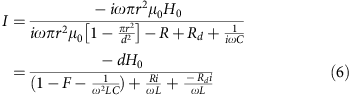Based on the magnetic moment per unit volume M=πr2I/d3 and μeff=(B/μ0)/(B/μ0M), we obtain the final effective permeability as follows: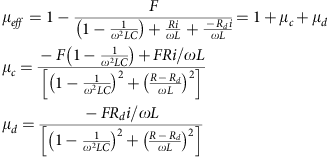Similar to the permittivity case, we find that the sign of the imaginary parts of μc and μd depends on the positive parasitic resistance R and the negative resistance –Rd of the TD. A negative imaginary part of μeff would appear when Rd>R, meanwhile the real part of μeff still exhibits a traditional Lorentzian dispersion.